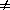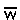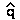The Mathematical Theory of Natural Selection

Charles Darwin described Natural Selection as an evolutionary process

If:     variation exists for some trait, and
fitness difference is correlated with that trait, and
trait is to some degree heritable (determined by genetics),
Then: trait distribution will change
over life history within single generation, and
between generations.

Process of change called "adaptation"

Or, "Natural Selection" is a process in which
"adaptation" occurs such that "fitness" increases
[Discourse on SVO structure of English language: 'adaptation' as noun & verb]

Under certain conditions, this results in Descent with Modification (evolution)
& The Origin of Species

The General Selection Model

Evolution & Natural Selection can be modeled genetically

Natural Selection results in change of allele frequency (q) [read "delta q"]
in consequence of differences in relative fitness (W)
of phenotypes to which alleles contribute.

Fitness is a phenotype of individual organisms (Darwinian fitness)
Fitness determined genetically (at least in part).
Fitness related to success at Survival AND Reproduction.
Fitness can be measured & quantified : Analysis of survivorship & fecundity schedule
relative fitness of phenotypes (genotypes) assigned numerical values

Consequences of natural selection depend on dominance of fitness:
Are "fitter" phenotypes due to dominant or recessive allele ?

Then, allele frequency change over time predicted by General Selection Model [see derivation]q = [pq] [(q)(W2 - W1) + (p)(W1 - W0)] /where W0, W1, & W2 are measure of fitness phenotypes
of AA, AB, & BB genotypes, respectively,read as "W bar" = Mean fitness

Consider simplest case:  Complete Dominance

genotype:   AA     AB      BB
phenotype: W0  =  WW2    (AA & AB phenotypes identical)

Model simplifies topq2(W2 - W1)     (since W1 - W0 = 0)as 'proportional to')

If 'B' phenotype more fit than 'A' phenotype,
W2 > W1   &q > 0    so   q increases

If 'B' phenotype less fit than 'A' phenotype,
W2 < W1    &q < 0    so   q decreases

then(W2 - W1) : greater difference in fitness,
greater intensity of selection
more rapid change

A numerical example of Selection:
Tay-Sachs Disease is caused by a series of alleles that are
rare            (q =  0.001)
recessive   (W0 = W1 = 1)
lethal         (W2 = 0)

Thenq = pq2(W2 - W1) = -pq-q2   (since if q << p, then p ~ 1)

That is, Natural Selection reduces frequency of Tay-Sachs allele
~ one part in a million (1 / 0.0012) per generation
q' = 0.001000 - 0.000001 = 0.000999

General cases of Directional Selection
Dependence on Dominance model [SR2019 4.3]
Rate ofvsq [SR2019 4.2]

Alternate notation with selection coefficients

s = 1 - W

Selection Coefficient (s) = difference in fitness
of phenotype relative to 'standard' phenotype with fitness  W = 1
Math simpler because only one variable used

(1) Complete dominance

genotype:   AA      AB     BB
phenotype:  W0 =  WW2    (AA and AB have identical phenotypes)
or        1   =   11 - s

if  0 < s < 1 : 'B' is deleterious (at a selective disadvantage)
if  s < 0       : 'B' is advantageous

thenq = -spq2 / (1 - sq2)      [see derivation]

(2) Incomplete dominance

genotype:    AA     AB        BB
phenotype:  WWW2    (all phenotypes different)
or           1 - s11 - s2

if 0 < s1 & s2 < 1 : "overdominance" of fitness (heterozygote advantage)
Population has optimal fitness when both alleles retained:
q will reach an equilibrium whereq = 0
0 << 1   (read as, "q hat")

then= (s1) / (s1 + s2)          [see derivation]

Other alternatives (Gillespie, 1968) [see SR2019 Table 4.4]

genotype:   AA      AB      BB
phenotype:    1     1 - hs   1 - s

1 - s = fitness difference between homozygotes
h scales relative fitness of heterozygote wrt homozygotes
if h = 1       then (1 - hs) = (1 - s)                 fitness of AB = BB
if h = 0      then (1 - hs) = 1                      fitness of AB = AA
if h = 0.5   then (1 - hs) = (1 - (0.5)(s))     fitness of AB intermediate bx AA & BB
semi-dominance
: each allele contributes equally to heterozygote fitness

HOMEWORK: what if 0 < h < 1 ?

The General Selection Model: Summary

Direction of allele frequency change due to fitness difference of alleles
(whether effect of allele on phenotype deleterious or advantageous).
Ultimate consequences depend on Dominance of Fitness [SR2019 4.2]
(whether allele dominant, semi-dominant, or recessive).
Rate of change: interplay of both factors (see MATLAB exercise),
q0 at start, if  q < or << 0.1) at start

AA   AB     BB    Consequence of natural selection   [ letq = change in f(B) ]

W0 =  W1 =  W2    No selection (neither allele has selective advantage):
thenq = 0,  H-W proportions remain constant

W0 =  W1 >  W2    deleterious recessive (= advantageous dominant):
thenq < 0,     q0.00  (loss): how fast? Does it get there?

W0 =  W1 <  W2    advantageous recessive (= deleterious dominant):
thenq > 0,     q1.00  (fixation): how fast?

W0 <  W1 >  W2    heterozygote superiority     [special case of incomplete dominance]:
AKA "overdominance", whereq = 0

Ex.: Balancing selection for Hemoglobin S & A allelesHOMEWORK

See National Public Radio story on societal aspects of Sickle-Cell Anemia

See National Public Radio story on use of CRISPR to treat Sickle-Cell Anemia

Alternative models of Natural Selection

Natural Selection in natural populations

Text material © 2022 by Steven M. Carr